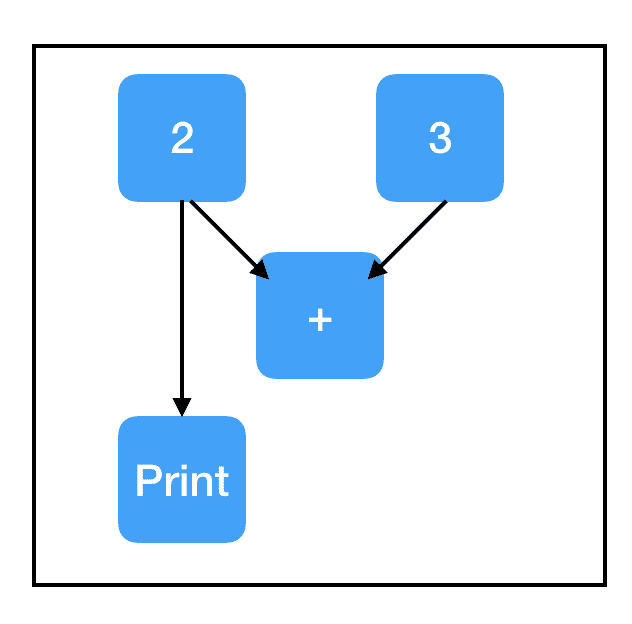Content Entry

## TensorFlow : The Confusing Parts 1 (中文)

Published: 2018-06-30 Categories: Algorithms Tags: Tensorflow

### 过去的教程缺少哪些内容？

Tensorflow 发布已经有三年，如今它已成为深度学习生态系统的基石。然而对于初学者来说它并不怎么简单易懂，与 PyTorch 或 DyNet 这样的运行即定义的神经网络库相比就更明显了。

### 理解 Tensorflow

Tensorflow 不是一个普通的 Python 库

Python 和 Tensorflow 之间的联系，可以类比 Javascript 和 HTML 之间的关系。Javascript 是一种全功能的编程语言，可以实现各种出色的效果。HTML 是用于表示某种类型的实用计算抽象（这里指的是可由 Web 浏览器呈现的内容）的框架。Javascript 在交互式网页中的作用是组装浏览器看到的 HTML 对象，然后在需要时通过将其更新为新的 HTML 来与其交互。

### 第一个关键抽象：计算图

``````# 代码
import tensorflow as tf
``````Code:

``````import tensorflow as tf
two_node = tf.constant(2)
print two_node
``````

Output:

``````Tensor("Const:0", shape=(), dtype=int32)
``````Code:

``````import tensorflow as tf
two_node = tf.constant(2)
another_two_node = tf.constant(2)
two_node = tf.constant(2)
tf.constant(3)
``````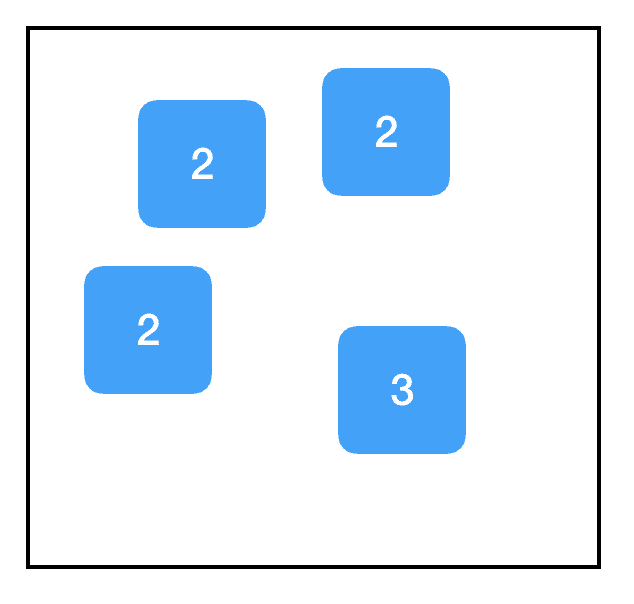Code:

``````import tensorflow as tf
two_node = tf.constant(2)
another_pointer_at_two_node = two_node
two_node = None
print two_node
print another_pointer_at_two_node
``````

Output:

``````None
Tensor("Const:0", shape=(), dtype=int32)
``````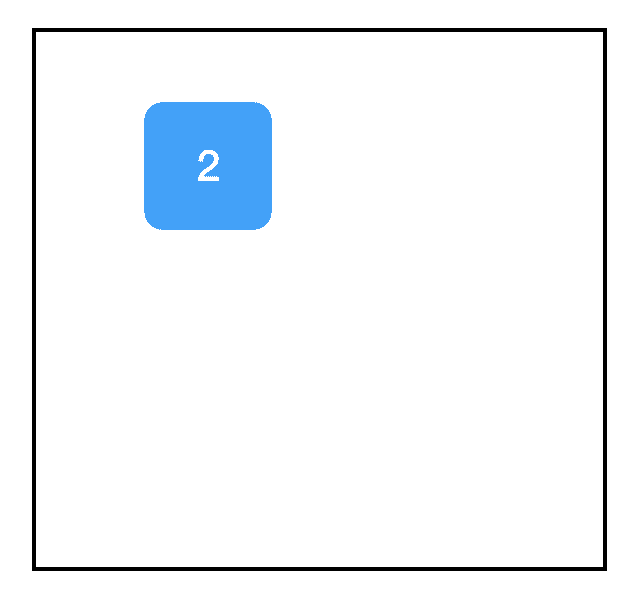Code:

``````import tensorflow as tf
two_node = tf.constant(2)
three_node = tf.constant(3)
sum_node = two_node + three_node ## equivalent to tf.add(two_node, three_node)
``````

Graph: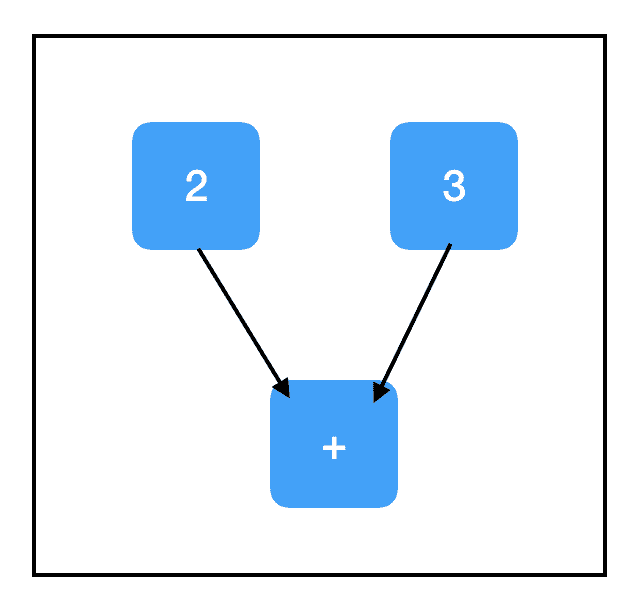### 第二个关键抽象：会话

Code:

``````import tensorflow as tf
two_node = tf.constant(2)
three_node = tf.constant(3)
sum_node = two_node + three_node
sess = tf.Session()
print sess.run(sum_node)
``````

Output:

``````5
``````

Graph:Code:

``````import tensorflow as tf
two_node = tf.constant(2)
three_node = tf.constant(3)
sum_node = two_node + three_node
sess = tf.Session()
print sess.run([two_node, sum_node])
``````

Output:

``````[2, 5]
``````

Graph:### 占位符和 feed_dict

Code:

``````import tensorflow as tf
input_placeholder = tf.placeholder(tf.int32)
sess = tf.Session()
print sess.run(input_placeholder)
``````

Output:

``````Traceback (most recent call last):
...
InvalidArgumentError (see above for traceback): You must feed a value for placeholder tensor 'Placeholder' with dtype int32
[[Node: Placeholder = Placeholder[dtype=DT_INT32, shape=<unknown>, _device="/job:localhost/replica:0/task:0/device:CPU:0"]()]]
``````

Graph:……这是个糟糕的例子，因为它引发了一个异常。占位符预计会被赋予一个值，但我们没有提供，因此 Tensorflow 崩溃了。

Code:

``````import tensorflow as tf
input_placeholder = tf.placeholder(tf.int32)
sess = tf.Session()
print sess.run(input_placeholder, feed_dict={input_placeholder: 2})
``````

Output:

``````2
``````

Graph:Code:

``````import tensorflow as tf
input_placeholder = tf.placeholder(tf.int32)
three_node = tf.constant(3)
sum_node = input_placeholder + three_node
sess = tf.Session()
print sess.run(three_node)
print sess.run(sum_node)
``````

Output:

``````3
Traceback (most recent call last):
...
InvalidArgumentError (see above for traceback): You must feed a value for placeholder tensor 'Placeholder_2' with dtype int32
[[Node: Placeholder_2 = Placeholder[dtype=DT_INT32, shape=<unknown>, _device="/job:localhost/replica:0/task:0/device:CPU:0"]()]]
``````

Graph: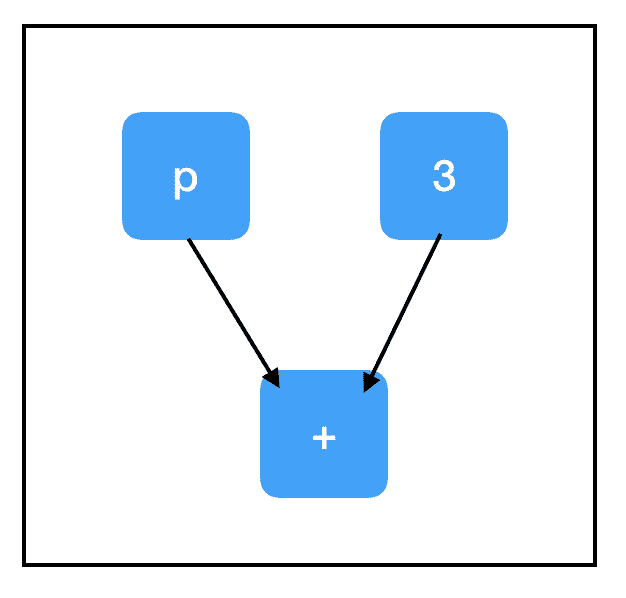Graph: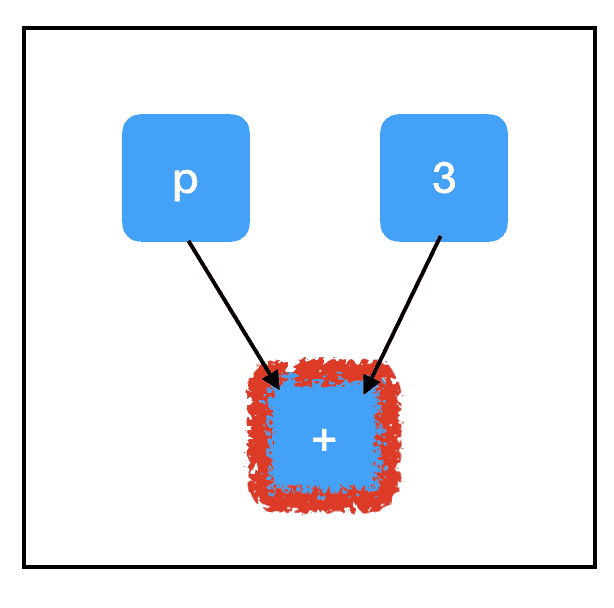Graph: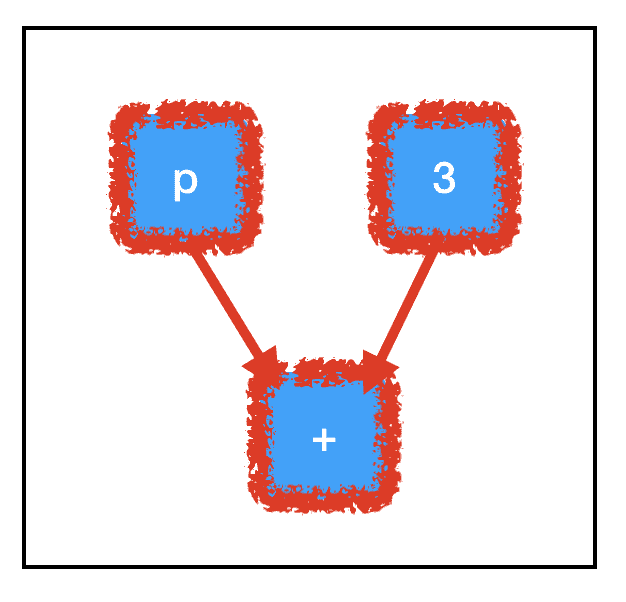Graph: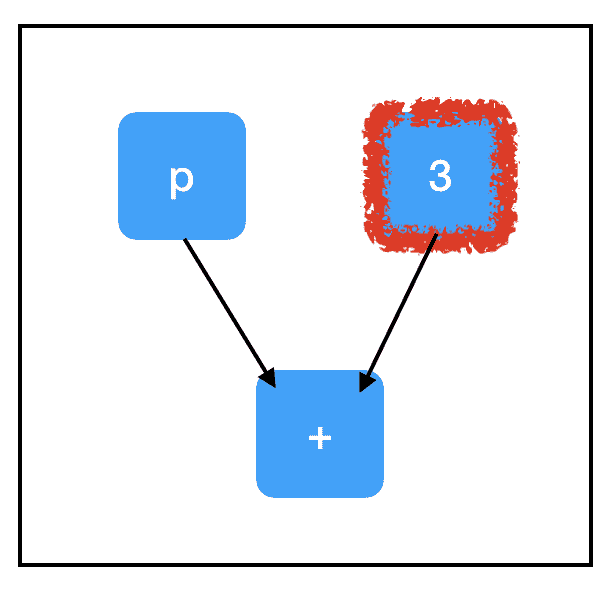Tensorflow 仅通过必需的节点自动路由计算这一事实是它的巨大优势。如果计算图非常大并且有许多不必要的节点，它就能节约大量运行时间。它允许我们构建大型的“多用途”图形，这些图形使用单个共享的核心节点集合根据采取的计算路径来做不同的任务。对于几乎所有应用程序而言，根据所采用的计算路径考虑 sess.run() 的调用方法是很重要的。

### 变量和副作用

Code:

``````import tensorflow as tf
count_variable = tf.get_variable("count", [])
sess = tf.Session()
print sess.run(count_variable)
``````

Output:

``````Traceback (most recent call last):
...
tensorflow.python.framework.errors_impl.FailedPreconditionError: Attempting to use uninitialized value count
[[Node: _retval_count_0_0 = _Retval[T=DT_FLOAT, index=0, _device="/job:localhost/replica:0/task:0/device:CPU:0"](count)]]
``````

Graph: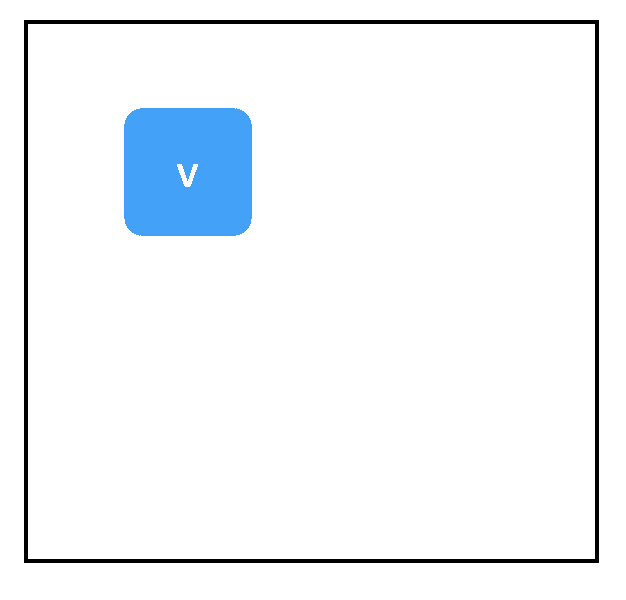Code:

``````import tensorflow as tf
count_variable = tf.get_variable("count", [])
zero_node = tf.constant(0.)
assign_node = tf.assign(count_variable, zero_node)
sess = tf.Session()
sess.run(assign_node)
print sess.run(count_variable)
``````

Output:

``````0
``````

Graph: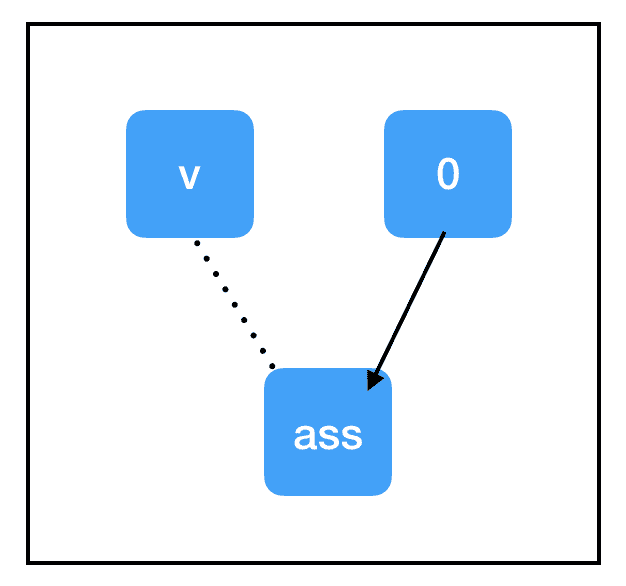• 标识操作。tf.assign(target,value) 不做任何计算，它总是与 value 相等。
• 副作用。当计算“流经”assign_node 时，就会给图中的其他节点带来副作用。在这种情况下，副作用就是用保存在 zero_node 中的值替换 count_variable 的值。
• 非依赖边。即使 count_variable 节点和 assign_node 在图中是相连的，两者都不依赖于其他节点。这意味着在计算任一节点时，计算不会通过该边回流。不过，assign_node 依赖 zero_node，它需要知道要分配什么。

“副作用”节点充斥在大部分 Tensorflow 深度学习工作流中，因此，请确保你对它们了解得一清二楚。当我们调用 sess.run(assign_node) 时，计算路径将经过 assign_node 和 zero_node。

Graph: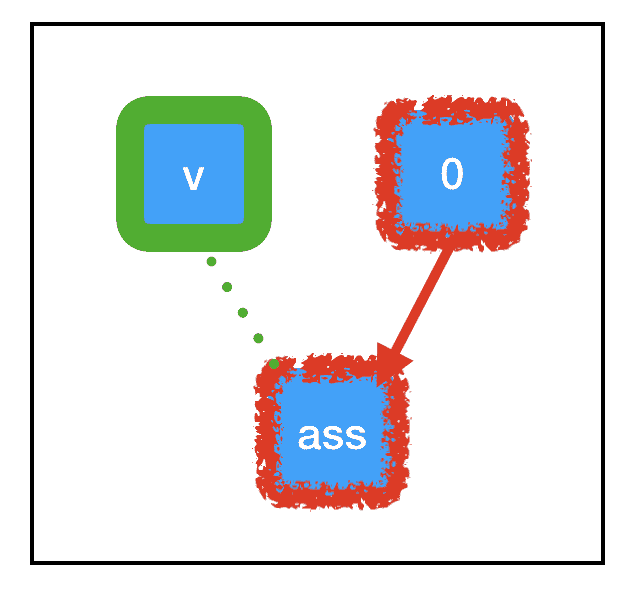Code:

``````import tensorflow as tf
const_init_node = tf.constant_initializer(0.)
count_variable = tf.get_variable("count", [], initializer=const_init_node)
sess = tf.Session()
print sess.run([count_variable])
``````

Output:

``````Traceback (most recent call last):
...
tensorflow.python.framework.errors_impl.FailedPreconditionError: Attempting to use uninitialized value count
[[Node: _retval_count_0_0 = _Retval[T=DT_FLOAT, index=0, _device="/job:localhost/replica:0/task:0/device:CPU:0"](count)]]
``````

Graph: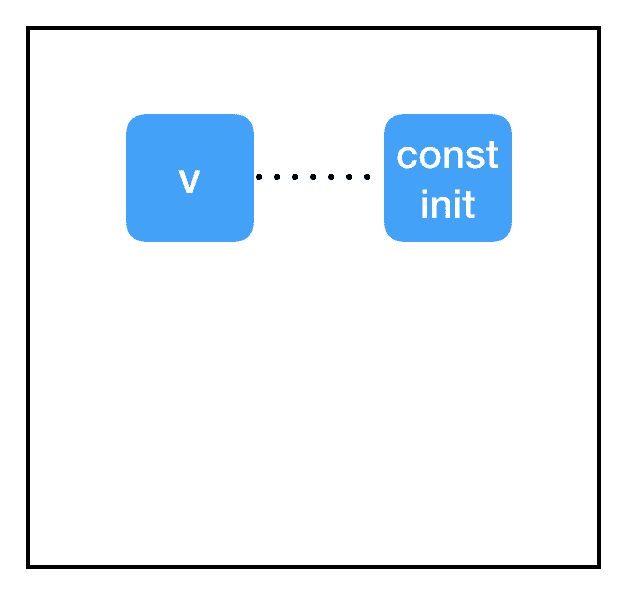Code:

``````import tensorflow as tf
const_init_node = tf.constant_initializer(0.)
count_variable = tf.get_variable("count", [], initializer=const_init_node)
init = tf.global_variables_initializer()
sess = tf.Session()
sess.run(init)
print sess.run(count_variable)
``````

Output:

``````0.
``````

Graph: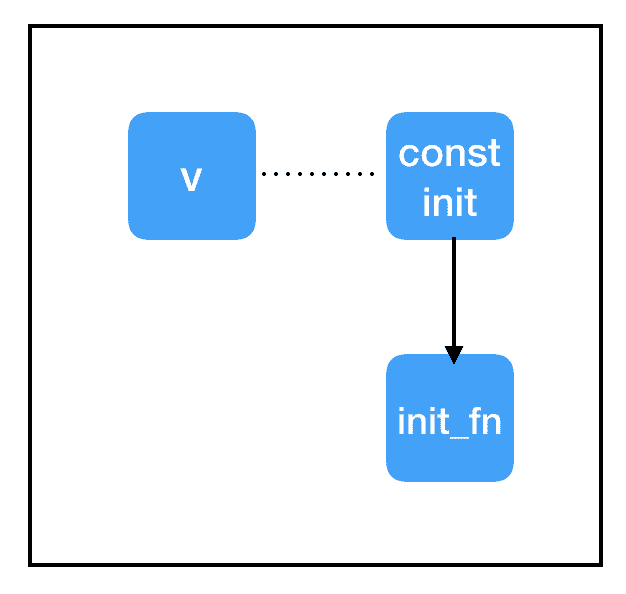### 在深度学习中，典型的“内循环”训练如下：

• 获取输入和 true_output
• 根据输入和参数计算出一个“猜测”
• 根据猜测和 true_output 之间的差异计算出一个“损失”
• 根据损失的梯度更新参数

Code:

``````import tensorflow as tf

### build the graph
## first set up the parameters
m = tf.get_variable("m", [], initializer=tf.constant_initializer(0.))
b = tf.get_variable("b", [], initializer=tf.constant_initializer(0.))
init = tf.global_variables_initializer()

## then set up the computations
input_placeholder = tf.placeholder(tf.float32)
output_placeholder = tf.placeholder(tf.float32)

x = input_placeholder
y = output_placeholder
y_guess = m * x + b

loss = tf.square(y - y_guess)

## finally, set up the optimizer and minimization node
train_op = optimizer.minimize(loss)

### start the session
sess = tf.Session()
sess.run(init)

### perform the training loop
import random

## set up problem
true_m = random.random()
true_b = random.random()

for update_i in range(100000):
## (1) get the input and output
input_data = random.random()
output_data = true_m * input_data + true_b

## (2), (3), and (4) all take place within a single call to sess.run()!
_loss, _ = sess.run([loss, train_op], feed_dict={input_placeholder: input_data, output_placeholder: output_data})
print update_i, _loss

### finally, print out the values we learned for our two variables
print "True parameters:     m=%.4f, b=%.4f" % (true_m, true_b)
print "Learned parameters:  m=%.4f, b=%.4f" % tuple(sess.run([m, b]))
``````

Output:

``````0 2.3205383
1 0.5792742
2 1.55254
3 1.5733259
4 0.6435648
5 2.4061265
6 1.0746256
7 2.1998715
8 1.6775116
9 1.6462423
10 2.441034
...
99990 2.9878322e-12
99991 5.158629e-11
99992 4.53646e-11
99993 9.422685e-12
99994 3.991829e-11
99995 1.134115e-11
99996 4.9467985e-11
99997 1.3219648e-11
99998 5.684342e-14
99999 3.007017e-11
True parameters:     m=0.3519, b=0.3242
Learned parameters:  m=0.3519, b=0.3242
``````

Code:

``````## finally, set up the optimizer and minimization node
train_op = optimizer.minimize(loss)
``````

train_op 回溯其输入的计算路径，寻找变量节点。对于找到的每个变量节点，它计算与损失相关的变量梯度。然后，它为该变量计算新值：当前值减去梯度乘以学习率。最后，它执行一个赋值操作来更新变量的值。

### 用 tf.Print 进行调试

Code:

``````import tensorflow as tf
two_node = tf.constant(2)
three_node = tf.constant(3)
sum_node = two_node + three_node
sess = tf.Session()
print sess.run(sum_node)
``````

Output:

``````3
``````

Code:

``````import tensorflow as tf
two_node = tf.constant(2)
three_node = tf.constant(3)
sum_node = two_node + three_node
sess = tf.Session()
answer, inspection = sess.run([sum_node, [two_node, three_node]])
print inspection
``````

Output:

``````[2, 3]
5
``````

Code:

``````import tensorflow as tf
two_node = tf.constant(2)
three_node = tf.constant(3)
sum_node = two_node + three_node
print_sum_node = tf.Print(sum_node, [two_node, three_node])
sess = tf.Session()
print sess.run(print_sum_node)
``````

Output:

``````
5
``````

Graph: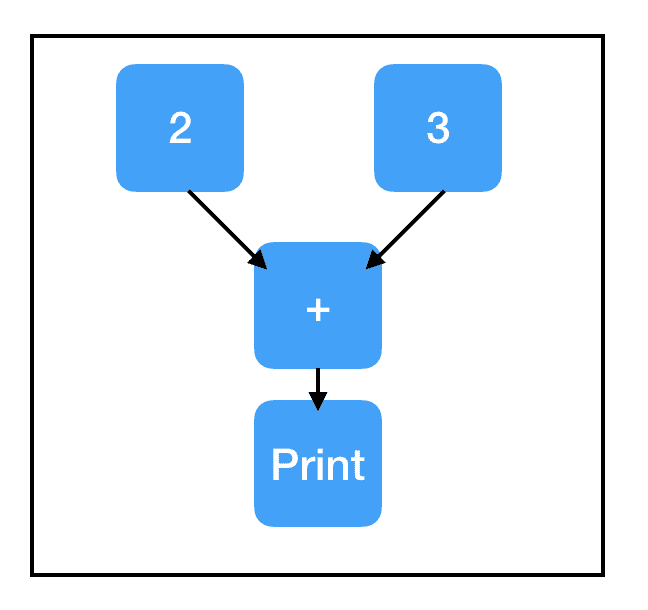Code:

``````import tensorflow as tf
two_node = tf.constant(2)
three_node = tf.constant(3)
sum_node = two_node + three_node
### this new copy of two_node is not on the computation path, so nothing prints!
print_two_node = tf.Print(two_node, [two_node, three_node, sum_node])
sess = tf.Session()
print sess.run(sum_node)
``````

Output:

``````5
``````

Graph: